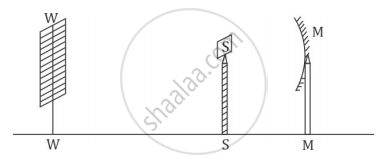# A student obtains a sharp image of the distant window (W) of the school laboratory on the screen (S) using the given concave mirror (M) to determine its focal length. - Science

MCQ

A student obtains a sharp image of the distant window (W) of the school laboratory on the screen (S) using the given concave mirror (M) to determine its focal length. Which of the following distances should he measure to get the focal length of the mirror?• MW

• MS

• SW

• MW-WS

#### Solution

MS

Explanation:

The focal length of a concave mirror is the distance between its pole and principal focus.

That is, the distance of the image formed (screen) from the concave mirror will be equal to the focal length of the concave mirror.

Concept: Spherical Mirrors
Is there an error in this question or solution?
2014-2015 (March) All India Set 1

Share